## Posts Tagged ‘C++’

### Triangle Identification – C++ solution and test code

Tuesday, September 23rd, 2008

Here is another interview coding question that I have heard of.

You have to write a function to identify the triangle type. You have to return a number between 1 or 4 to identify either a valid triangle type or an error.

The solution is very simple requiring only one condensed line of code to identify each triangle type. Two lines of code identify error cases. One of the error cases identifies input with 0 or negative lengths. The other error case identifies line lengths that don’t make a triangle or which make a segment (thanks to Yabba for pointing out these error cases).

As an exercise you could try to extend the solution to identify right-angled triangles as well.

```const int SCALENE = 1;
const int ISOSCELES = 2;
const int EQUILATERAL = 3;
const int ERROR = 4;
int TriangleType(int x, int y, int z)
{
if ((x + y <= z) || (x + z <= y) || (z + y <= x)) return ERROR;
if (x<=0 || y<=0 || z<=0) return ERROR;
if (x == y && y == z) return EQUILATERAL;
if (x == y || y == z || z == x) return ISOSCELES;
return SCALENE;
}
```

The more interesting code comes from testing the function. To test the function I pass in a series of triangle side lengths. I also pass in the expected result. If the actual result matches the expected result then the test passes.

The test data is written in a way that allows each test to be defined in a single line of code which makes it very easy to extend.

This easy extendibility was fortunate as my original version of this solution missed out the cases where the line lengths don’t make a triangle (e.g. 1, 1, 5), and where the line lengths make a segment (e.g. 2, 2, 4).

```void TriangleTest()
{
const int testDataSize = 14;
int testData[testDataSize] = {
{9, 9, 9, EQUILATERAL},
{4, 5, 5, ISOSCELES},
{5, 4, 5, ISOSCELES},
{5, 3, 3, ISOSCELES},
{0, 0, 0, ERROR}, // 0 number checks
{0, 1, 2, ERROR},
{1, 0, 2, ERROR},
{1, 2, 0, ERROR},
{-1, -1, -1, ERROR}, // negative number checks
{-1, 1, 2, ERROR},
{1, -1, 2, ERROR},
{1, 2, -1, ERROR},
{2, 2, 4, ERROR}, // line segment not a triangle
{1, 1, 5, ERROR} // not a triangle
};
for (int i=0; i<testDataSize; ++i)
{
int result = TriangleType(
testData[i], testData[i], testData[i]);
if (result == testData[i])
{
cout << " Pass: triangleType("
<< testData[i] << ", "
<< testData[i] << ", "
<< testData[i] << ")==" << result << endl;
}
else
{
cout << "!Fail: triangleType("
<< testData[i] << ", "
<< testData[i] << ", "
<< testData[i] << ")==" << result
<< " Expected: " << testData[i] << endl;
}
}
}
```

### Reverse the words in a sentence – C++ solution and test code

Tuesday, September 16th, 2008

Here is a solution to the standard interview questions of reversing the letters in the words of a sentence. It is a more complex version of the even more common “reverse a string” question. I also include my test code.

There are two main parts to this. The first identifies where the word boundaries are. The second reverses the letters between two positions in the array.

The wordStart and wordEnd variables keep track of the word boundaries. We first look for the start of the word and then the end and store the positions. These values are then passed to ReverseWord along with the character array. ReverseWord does some simple character swapping to reverse the word.

```void ReverseWords(char string[])
{
int length = StringLength(string);
int wordStart = -1;
int wordEnd = -1;
for (int i=0; i<length; ++i)
{
if (string[i] == ' ' && wordStart == -1)
{
continue;
}
else if (wordStart == -1)
{
wordStart = i;
}
if (wordStart != -1 && string[i] == ' ')
{
wordEnd = i-1;
ReverseWord(string, wordStart, wordEnd);
wordStart = -1;
wordEnd = -1;
}
}
if (wordStart != -1)
{
wordEnd = length-1;
ReverseWord(string, wordStart, wordEnd);
}
}
void ReverseWord(char string[], int wordStart, int wordEnd)
{
int midPoint = (wordStart+wordEnd)/2;
for (int l=wordStart, r=wordEnd; l<=midPoint; ++l, --r)
{
char tmp = string[l];
string[l] = string[r];
string[r] = tmp;
}
}
```

Here is the test code. For each test a character array is passed to the word reversing function. The result is then compared against the expected result. If they match the test passes. If they don’t match then the test fails. I’ve tried to test the obvious cases. You could easily find flaws in the word reversal function but it is probably good enough to get you onto the next question.

```void ReverseWordsTest()
{
char test1[] = "cat and dog";
char expected1[] = "tac dna god";
ReverseAndCheck(test1, expected1);
char test2[] = "cat and dog ";
char expected2[] = "tac dna god ";
ReverseAndCheck(test2, expected2);
char test3[] = " cat and dog";
char expected3[] = " tac dna god";
ReverseAndCheck(test3, expected3);
char test4[] = " cat and dog ";
char expected4[] = " tac dna god ";
ReverseAndCheck(test4, expected4);
char test5[] = "cat  and dog";
char expected5[] = "tac  dna god";
ReverseAndCheck(test5, expected5);
char test6[] = "catanddog";
char expected6[] = "goddnatac";
ReverseAndCheck(test6, expected6);
char test7[] = "";
char expected7[] = "";
ReverseAndCheck(test7, expected7);
}
void ReverseAndCheck(char string[], char expected[])
{
ReverseWords(string);
if (MatchingStrings(string, expected))
{
cout << " Pass: " << string << endl;
}
else
{
cout << "!Fail: " << string << " != " << expected << endl;
}
}
bool MatchingStrings(char string1[], char string2[])
{
int length = StringLength(string1);
if (length != StringLength(string2))
{
return false;
}
for (int i=0; i<length; ++i)
{
if (string1[i] != string2[i])
{
return false;
}
}
return true;
}
int StringLength(char string[])
{
int index = 0;
char s = string[index];
while (s)
{
++index;
s = string[index];
}
return index;
}
```

### Picking the 5th from last element in a singly linked list

Monday, August 18th, 2008

Here’s another of those fun coding interview puzzles. The kind that companies like Microsoft or Google might ask you. Given a singly linked list, select the 5th from last element. I’m writing the solution in C++ but the solution in Java would be almost identical. I’m also putting up my test code.

The restrictions are – you can only make one pass of the list, and you don’t know the length of the list.

First we need to define our linked list element. All it needs is to store a value, and a pointer to the next element in the list.

```class Element
{
public:
Element(int valueArg) : value(valueArg), next(NULL) {}
public:
int value;
Element* next;
};
```

Here is the actual solution. As we are only allowed one pass of the list we use two pointers. The second pointer trails 5 places behind the first pointer. When the first pointer reaches the end of the list we simply return whichever element the second pointer is pointing to.

We need to take care of the special case where the list has less than 5 elements – including the case where the list has 0 elements!

```Element* FifthFromLast(Element* root)
{
Element* current = root;
int fromLast = 5;
while (fromLast > 0 && current)
{
current = current->next;
--fromLast;
}
if (fromLast != 0)
{
return NULL; // less than 5 items in list
}
Element* fifthFromLast = root;
while (current)
{
current = current->next;
fifthFromLast = fifthFromLast->next;
}
return fifthFromLast;
}
```

Here is the test code which tests the most common cases for this problem. As the parameter to FifthFromLast is the root element we can simulate a shorter list by passing in one of the middle elements.

```void LinkedListTest()
{
Element* one = new Element(1);
Element* two = new Element(2);
Element* three = new Element(3);
Element* four = new Element(4);
Element* five = new Element(5);
Element* six = new Element(6);
Element* seven = new Element(7);
Element* eight = new Element(8);
Element* nine = new Element(9);
Element* ten = new Element(10);
one->next = two;
two->next = three;
three->next = four;
four->next = five;
five->next = six;
six->next = seven;
seven->next = eight;
eight->next = nine;
nine->next = ten;
cout << "Test: find 5th from last in 10 item list" << endl;
Element* fifthFromLast = FifthFromLast(one);
if (fifthFromLast == six)
{
cout << " Pass" << endl;
}
else
{
cout << "!Fail" << endl;
}
cout << "Test: with 4 item list - expect NULL" << endl;
Element* nullReturnCheck = FifthFromLast(seven);
if (nullReturnCheck == NULL)
{
cout << " Pass" << endl;
}
else
{
cout << "!Fail" << endl;
}
cout << "Test: FifthFromLast(NULL) - expect NULL" << endl;
nullReturnCheck = FifthFromLast(NULL);
if (nullReturnCheck == NULL)
{
cout << " Pass" << endl;
}
else
{
cout << "!Fail" << endl;
}
// clean up
Element* current = one;
while (current)
{
Element* next = current->next;
delete current;
current = next;
}
}
```

This is the output from running the tests.

```Test: find 5th from last in 10 item list
Pass
Test: with 4 item list - expect NULL
Pass
Test: FifthFromLast(NULL) - expect NULL
Pass
```

### Reverse a linked list – C++ source code

Monday, July 21st, 2008

Reversing a linked list is a simple programming problem, which is often an interview question. In this case I’m referring to a singly linked list. I’ll provide the C++ solution and the C++ test code.

One of the easiest ways to reverse the linked list is to create a new head pointer, iterate through the list removing each item in turn and then inserting them at the beginning of the new list.

Here is the definition of my linked list node and the code to reverse the list.

```class Node
{
public:
Node(int value) : value(value), next(NULL) {}
public:
int value;
Node* next;
};
{
Node* newList = NULL;
while (current)
{
Node* next = current->next;
current->next = newList;
newList = current;
current = next;
}
return newList;
}
```

Below is the code I used to test this. This is what it does:

1. It creates a simple linked list.
2. Prints it out – output should be ‘12345’.
3. Reverses it.
4. Prints it out – output should be ‘54321’.
5. Reverses it again.
6. Prints it – output should be ‘12345’.
7. Deletes the list.
```void listTests()
{
Node* one = new Node(1);
Node* two = new Node(2);
Node* three = new Node(3);
Node* four = new Node(4);
Node* five = new Node(5);
one->next = two;
two->next = three;
three->next = four;
four->next = five;
// cleanup memory
while (current)
{
Node* next = current->next;
delete current;
current = next;
}
}
{
while (current)
{
cout << current->value;
current = current->next;
}
cout << endl;
}
```

This should be the output:

```12345
54321
12345
```

If you are interested in other linked list related code I have a post about picking the 5th from last element from a singly linked list, and one on selecting random numbers from a stream or linked list. Both of these are based on programming interview questions.

### Dijkstra’s Algorithm code in C++

Tuesday, July 15th, 2008

Dijkstra’s algorithm is a famous algorithm that calculates the routes and distances from a start node to all other nodes in a connected graph where all the distances are positive.

Here is a version which I wrote in C++. My aim here was to try to create a version that was easy to read, rather than the most efficient version. I used the excellent Introduction to Algorithms book as a reference for my version of Dijkstra’s. This version is implemented using STL vectors to store the edges and nodes. I might later modify it to use adjacency lists and priority queues to produce a more efficient implementation.

First off is my `Node` and `Edge` class. You’ll note that the objects add themselves to the `nodes` and `edges` vectors as they are constructed.

```vector<Node*> nodes;
vector<Edge*> edges;
class Node
{
public:
Node(char id)
: id(id), previous(NULL),
distanceFromStart(INT_MAX)
{
nodes.push_back(this);
}
public:
char id;
Node* previous;
int distanceFromStart;
};
class Edge
{
public:
Edge(Node* node1, Node* node2, int distance)
: node1(node1), node2(node2), distance(distance)
{
edges.push_back(this);
}
bool Connects(Node* node1, Node* node2)
{
return (
(node1 == this->node1 &&
node2 == this->node2) ||
(node1 == this->node2 &&
node2 == this->node1));
}
public:
Node* node1;
Node* node2;
int distance;
};
```

Next up is my code to construct a simple test graph. The starting node is initialised with a distance of 0. Dijkstra’s algorithm is then called before the results are printed out. Underneath the code is a diagram of what this graph looks like.

```void DijkstrasTest()
{
Node* a = new Node('a');
Node* b = new Node('b');
Node* c = new Node('c');
Node* d = new Node('d');
Node* e = new Node('e');
Node* f = new Node('f');
Node* g = new Node('g');
Edge* e1 = new Edge(a, c, 1);
Edge* e2 = new Edge(a, d, 2);
Edge* e3 = new Edge(b, c, 2);
Edge* e4 = new Edge(c, d, 1);
Edge* e5 = new Edge(b, f, 3);
Edge* e6 = new Edge(c, e, 3);
Edge* e7 = new Edge(e, f, 2);
Edge* e8 = new Edge(d, g, 1);
Edge* e9 = new Edge(g, f, 1);
a->distanceFromStart = 0; // set start node
Dijkstras();
PrintShortestRouteTo(f);
// Node / Edge memory cleanup snipped
}
```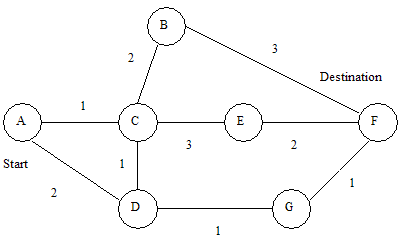Here is the actual algorithm implementation. We remove the node with the smallest distance from the list of nodes, and then calculate all the minimum distances. We repeat until there are no more nodes left.

```void Dijkstras()
{
while (nodes.size() > 0)
{
Node* smallest = ExtractSmallest(nodes);
for (int i=0; i<size; ++i)
{
int distance = Distance(smallest, adjacent) +
smallest->distanceFromStart;
{
}
}
}
}
```

Here are the supporting functions. The first function removes and returns the node with the smallest distance from the list.

The next returns a new vector containing all the nodes which are adjacent to the node that we pass in.

The third returns the distance value of an edge which directly connects the two nodes.

The final one checks if a node is in the list of nodes.

```// Find the node with the smallest distance,
// remove it, and return it.
Node* ExtractSmallest(vector<Node*>& nodes)
{
int size = nodes.size();
if (size == 0) return NULL;
int smallestPosition = 0;
Node* smallest = nodes.at(0);
for (int i=1; i<size; ++i)
{
Node* current = nodes.at(i);
if (current->distanceFromStart <
smallest->distanceFromStart)
{
smallest = current;
smallestPosition = i;
}
}
nodes.erase(nodes.begin() + smallestPosition);
return smallest;
}
// Return all nodes adjacent to 'node' which are still
// in the 'nodes' collection.
{
const int size = edges.size();
for(int i=0; i<size; ++i)
{
Edge* edge = edges.at(i);
if (edge->node1 == node)
{
}
else if (edge->node2 == node)
{
}
{
}
}
}
// Return distance between two connected nodes
int Distance(Node* node1, Node* node2)
{
const int size = edges.size();
for(int i=0; i<size; ++i)
{
Edge* edge = edges.at(i);
if (edge->Connects(node1, node2))
{
return edge->distance;
}
}
return -1; // should never happen
}
// Does the 'nodes' vector contain 'node'
bool Contains(vector<Node*>& nodes, Node* node)
{
const int size = nodes.size();
for(int i=0; i<size; ++i)
{
if (node == nodes.at(i))
{
return true;
}
}
return false;
}
```

And finally here is the code which prints out the route between our start node ‘a’ and the destination node ‘f’. It starts at the destination node and then works backwards using the previous pointer in each node.

```void PrintShortestRouteTo(Node* destination)
{
Node* previous = destination;
cout << "Distance from start: "
<< destination->distanceFromStart << endl;
while (previous)
{
cout << previous->id << " ";
previous = previous->previous;
}
cout << endl;
}
```

Update: 7th September 2008

I have now uploaded a zip file containing the Visual C++ Express Solution file and source code for this project – Dijkstra’s Source Code (8kb).

### Select 100 random values from a stream / linked list

Friday, July 11th, 2008

Here’s an interesting puzzle / interview question that I heard recently.

Problem

You have a either a linked list or a stream containing int values. You don’t know how large the stream / linked list except that it contains 100 or more values. You need to write a method that selects 100 random values from the stream. You have to write the method so that there isn’t a bias towards values at the start, middle or end of the stream / list. In other words the chances of picking a value from anywhere in the stream / list should be roughly the same.

There are some restrictions.
You can’t cache the whole stream (if it is a stream).
Or if it is a linked list – you are only allowed a single pass of the list. You can’t iterate through it once to determine its length.

Solution

The solution is:

1. Select the first 100 values and store them in an array.
2. For each value after the first 100 we select them according to a decreasing probability. If we select a value then we replace a randomly selected value from our existing list.

So values 1-100 have a probability of 1 of being initially selected.
Value 101 has a 100/101 chance of being selected.
Value 102 has a 100/102 chance of being selected.
Value 1050 has a 100/1050 chance… etc.

100 is at the top of the division as this is our value for x – the number of values we are selecting.

You can see that value 101 has a very high chance of being selected. 100/101 in fact. As we are replacing one of our existing values, each of the existing values has a 100/101 chance of remaining after the replacement.

Using this simple logic we can get a random sample of the values even though we don’t know the length of the stream / list.

Code

Here is the C++ code showing how to do this. Also included is a simple test which sends the values 1-1 million to the function. 100 are randomly selected.

After the code is a graph which shows the distribution of the selected values. You can see that the distribution is fairly even showing that the code works. For random number selection code such as this, the easiest way to verify that the code works is to manually view the results in a graph and see if the output looks sensible.

```const int size = 100;
int selected[size];
int count = 0;
void SelectRandomNumbers(int nextNum)
{
if (count < size)
{
selected[count++] = nextNum;
return;
}
double probability = (double)size / (double)(count+1);
double select = (double)rand() / RAND_MAX;
if (select < probability)
{
int randomIndex = RandomNumber(0, size);
selected[randomIndex] = nextNum;
}
++count;
}
// min is inclusive
// max is non-inclusive
int RandomNumber(int min, int max)
{
return (int)((double)rand()	/
(RAND_MAX + 1) * (max - min) + min);
}
///////////////

const int iterations = 1000000;
void RandomSelectionTest()
{
srand((unsigned)time(0));
for (int i=0; i<iterations; ++i)
{
SelectRandomNumbers(i);
}
cout << size <<	" randomly selected ints:" << endl;
PrintIntArray(selected, size);
}
void PrintIntArray(int thearray[], int size)
{
for (int i=0; i<size; ++i)
{
cout << thearray[i] << endl;
}
}
```

Graph

Here are the results. 1 million numbers were passed in. 100 were selected. You can see that the distribution is fairly even which means that the code is doing the correct thing.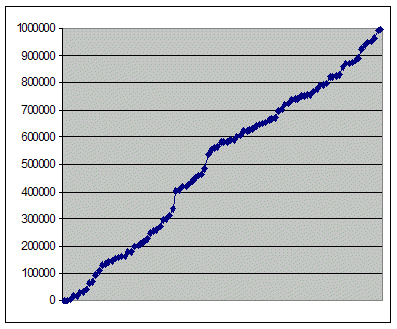### QuickSort Implementation Code in C++

Wednesday, June 25th, 2008

I was having a look at the QuickSort algorithm (as you do for fun) and was trying to find a tidy looking implementation in C++. I found a lot of implementations out there but most of them looked far more complicated than I thought they should have been.

The QuickSort algorithm as printed in the Introduction to Algorithms book looks relatively simple. I wanted a C++ version that has a QuickSort function and a partition function. Many of the implementations that I’ve seen on the internet have both functions merged into one.

The neatest looking QuickSort code that I found was one this Java version written by a Prof. Dr. H. W. Lang. Compare how short and easy to read this piece of code is to other QuickSort implementations. This is close to what I want but the partition part is rolled into the main QuickSort code.

It also had quite a few differences to the Introduction to Algorithms QuickSort. I used the Prof. Dr. H. W. Lang code as a base, split the partition part out, and then made a few other changes to make the implementation closer to the Introduction to Algorithms version. This is what I ended up with.

```int partition(int a[], int left, int right, int pivotIndex)
{
int pivot = a[pivotIndex];
do
{
while (a[left] < pivot) left++;
while (a[right] > pivot) right--;
if (left < right && a[left] != a[right])
{
swap(a[left], a[right]);
}
else
{
return right;
}
}
while (left < right);
return right;
}
void quicksort(int a[], int left, int right)
{
if (left < right)
{
int pivot = (left + right) / 2; // middle
int pivotNew = partition(a, left, right, pivot);
quicksort(a, left, pivotNew - 1);
quicksort(a, pivotNew + 1, right);
}
}```

I tested this in the free Microsoft Visual C++ Express Edition. Here is what I used to test the QuickSort code.

```#include "stdafx.h"
#include <iostream>
using namespace std;
void printArray(int thearray[], int count)
{
for (int i=0; i<count; ++i)
{
cout << thearray[i] << " ";
}
cout << endl;
}
void arraySortingTests()
{
// unique unsorted
int numbers1[] = {14, 17, 11, 13, 19, 16, 12, 15, 18, 10};
int numbers2[] = {19, 16, 10, 12, 14, 17, 13, 18, 15, 11};
int numbers3[] = {10, 11, 12, 13, 14, 15, 16, 17, 18, 19};
// reverse sorted
int numbers4[] = {19, 18, 17, 16, 15, 14, 13, 12, 11, 10};
// all duplicates
int numbers5[] = {7, 7, 7, 7, 7};
// 1 pair
int numbers6[] = {14, 17, 11, 13, 19, 16, 12, 15, 18, 14};
// 1 pair at start
int numbers7[] = {14, 14, 17, 11, 13, 19, 16, 12, 15, 18};
// multiple duplicates
int numbers8[] = {14, 14, 14, 13, 13, 13, 13, 12, 12, 12};
int numbers9[] = {12};
int numbers10[] = {12,10};
quicksort(numbers1, 0, 9);
printArray(numbers1, 10);
quicksort(numbers2, 0, 9);
printArray(numbers2, 10);
quicksort(numbers3, 0, 9);
printArray(numbers3, 10);
quicksort(numbers4, 0, 9);
printArray(numbers4, 10);
quicksort(numbers5, 0, 4);
printArray(numbers5, 5);
quicksort(numbers6, 0, 9);
printArray(numbers6, 10);
quicksort(numbers7, 0, 9);
printArray(numbers7, 10);
quicksort(numbers8, 0, 9);
printArray(numbers8, 10);
quicksort(numbers9, 0, 0);
printArray(numbers9, 1);
quicksort(numbers10, 0, 1);
printArray(numbers10, 2);
}
int _tmain(int argc, _TCHAR* argv[])
{
arraySortingTests();
return 0;
}
```

Update: 25/09/2011 as people noticed my original version of the Quick Sort algorithm didn’t work with duplicates. This new version does, and has extra tests cases to verify this. I’ve had to delete the comments which refer to the old version of the code, as they don’t make sense any more! If you spot any problems with this new version of the code, please let me know.
Update: 30/09/2011 Sergiy in the comments below has found a test case that this doesn’t work with :( If anyone can spot the fix please leave a comment.

### Writing and releasing a free software tool in two weeks

Tuesday, May 27th, 2008

Two weeks ago I started a mini-project to write a simple piece of software in C#, package it up and release it. Although I had initially given myself two weeks, the actual time I spent on this project was less than a week due to a pest problem, a birthday, and a family visit. The purpose of this project was to learn the basics of a new programming language, and to learn how to package and release the initial version of a new piece of simple software.

Picking a language

I had never written a line of C# in my life but knew that it had similarities to both C++ and Java. I’ve been programming with C++ and Java professionally (by which I mean that someone pays me to do it) for many years so it seemed an interesting new language to experiment with.

I downloaded the free Microsoft Visual C# 2008 Express Edition and installed it. I followed the initial tutorial to learn how to build a very basic application and then started experimenting with adding new features.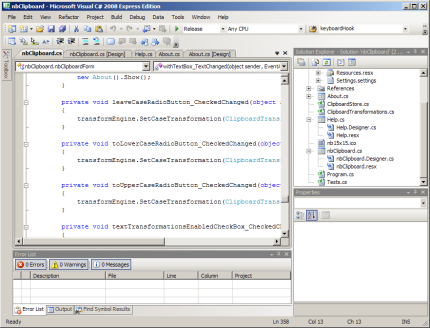Version control

The version control system that I am used to working with is Perforce. I therefore wanted to use a different version control system for this project (remember this is a learning exercise so I want to try new technologies!).

I picked the well known subversion as the version control tool with TortoiseSVN as the front end to subversion. Subversion is a popular choice and is easy to use if you are used to using something like Perforce. It has many differences but also many similarities.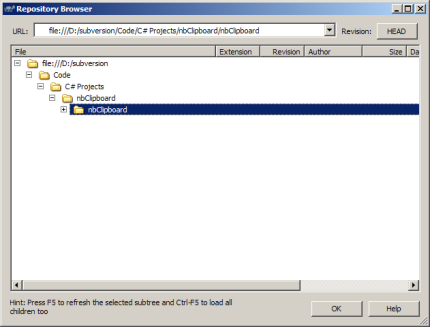What to write?

I had decided to write a Windows clipboard extender. There are loads of other clipboard extenders available but that didn’t worry me. My goal isn’t to create something original, just something that I would use myself.

The core features that I wanted to include was stack and queue support for the clipboard. Normally copying an item of text to the clipboard overwrites the previous item. I wanted the previous values to be stored in either a queue or a stack. e.g.

I copy (using Ctrl-C) the values ‘one’, ‘two’, ‘three’.
I then paste (using Ctrl-V) and the pasted values are ‘one’, ‘two’ and then ‘three’. This is the queue behaviour.
If using the stack the pasted values would be ‘three’, ‘two’ and finally ‘one’.

As I was completely new to C# and its APIs, I had to do a lot of searching to find out how to do Clipboard operations. The Visual C# help system was also very good.

There is a huge amount of help for almost any C# problem you can think of on the internet so it wasn’t hard to find out how to solve any problem that I had. In particular the following articles were very helpful:

Writing a EULA

When releasing software you would normally ship an end-user licence agreement (EULA) along with the software. This tell the user what they can and can’t do with your software.

If you want to open source your software there is a good source of licensing information at the Free Software Foundation.

In my case I wanted the software to be free to use but proprietary (I’m not shipping the source). It is harder to find information on how to produce a licence in this case. One option is to look at existing licences and adapt any bits you like. I used the XS EULA Generator (update: sadly the tool no longer exists) and then adapted it for my needs.

Creating an install package

Once I had written my clipboard extender (which I named nbClipboard) I needed some way to package it up with a Windows installer.

After looking around the best option seemed to be Inno Setup combined with ISTool (a front end for Inno Setup). You can download both together by going to the Inno Setup website and downloading the QuickStart Pack.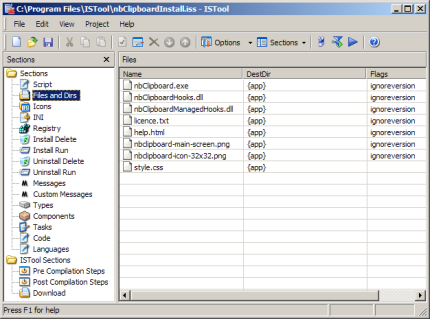This creates a professional looking software install executable which looks identical to the install tools used by many popular software applications.The format was created by the Association of Shareware Professionals (ASP) and they have a free PADGen tool that you can use to create the PAD file.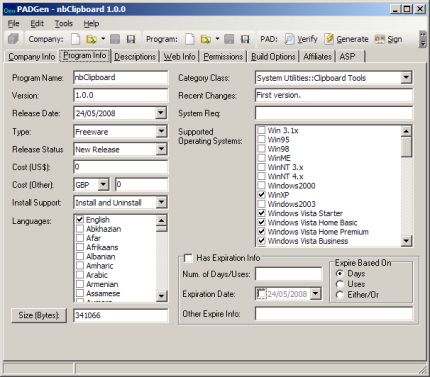Releasing the software

After creating the install package and the PAD file I uploded both of them to my website and then submitted the PAD file to the ASP repository (a store of PAD files that many software download sites use as a data source).

nbClipboard

nbClipboard is available from the below link and it might be available from some small software download sites as well – if you can find them!

nbClipboard Clipboard Extender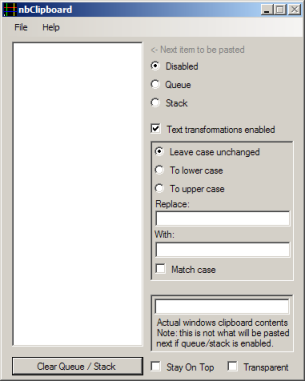It certainly won’t win any awards but then I was more interested in going through the whole process of writing and releasing a new software tool than creating something which was ground breaking.

### C# – How to make a window stay on top and become transparent

Sunday, May 18th, 2008

Whilst looking for information on how to make a window stay on top using C#, I spotted some odd solutions on a number of other web sites. After looking through the Form properties in Visual Studio C# 2008 myself it turns out that it is really easy to make a window stay on top. I added a check box to by application and when it is ticked the window stays on top.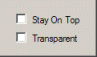```private void stayOnTopCheckBox_CheckedChanged(object sender,
EventArgs e)
{
if (stayOnTopCheckBox.Checked)
{
this.TopMost = true;
}
else
{
this.TopMost = false;
}
}
```

Along the same theme of ‘very cheap and quick tricks that you add to your C# program’ I added another box to make the window transparent. Below is the code and the result of ticking this box.

```private void transparentCheckBox_CheckedChanged(object sender,
EventArgs e)
{
if (this.transparentCheckBox.Checked)
{
this.Opacity = 0.5;
}
else
{
this.Opacity = 1;
}
}
```